Linear Transformations: Matrix Operations on Polyhedra

Here we write a function that will take a matrix and operate on all the points (i.e., position vectors) of all the triangles (The function uses a pattern for the arguments to Polygon, and then matrix multiplies the matches). The function will also take some text to help us visually identify which linear operation has been performed.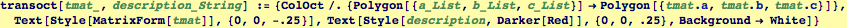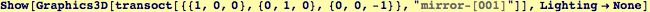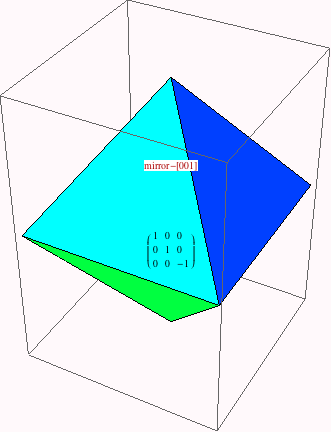We build a set of octahedra with different types of linear operations. We will visualize them in a few steps.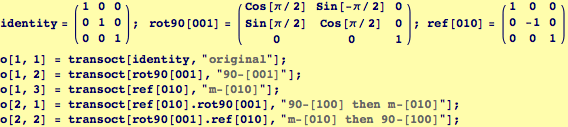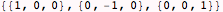Mathematica has many common geometric transformations available as functions: Here, we extract information about counter-clockwise rotation about .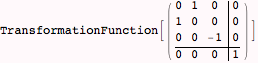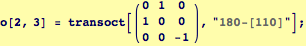We use Manipulate and GraphicsGrid to visualize the effect of all the transformations.  We use Manipulate's sliders to set the view point for all images simultaneously. To do this, we right a function that will place the viewpoint someplace on a sphere of radius 3.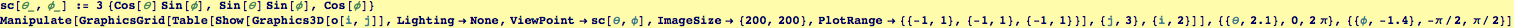Created by Wolfram Mathematica 6.0  (06 September 2007)